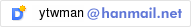# Luminance and Incidence angle question

Dear all

I’m worked Luminance analysis.

but, I saw Rivero formula. so I have question.

According to Rivero formula varies transmittance of the incidence angle.

Therefore, it seems to change the reflectance&transmittance of the incidence angle.

[Rivero formula, T1 = 1.018T2(Cosθ+sin^3θcosθ), T1= transmittance of the incidence angleθ, T2= transmittance of the perpendicularly incident]

According to my results

• Simulation summary -

[Glass material from optics6(NFRC ID 6974+6957), transmittance 0.5022, Reflectance(front) 0.178]

[Date : June 21, 19:05 , Incidence angle[deg] : 83.244 ]

[Latitude(deg) : 37.225 , Longitude(deg) : 127.07]

[Sky : clear sky ( +s)]

• Simulation result-

Sun Luminance : 295,473,152 cd/m2

A spot Lumimance(Specular reflection) : 52,734,259 cd/m2 | B spot Luminance(Specular reflection) : 52,734,259 cd/m2

A spot/Sun : 0.1785 , B spot/Sun : 0.1785

This Reflectance ratio is similar of the glass material.

I did the simulation proceeds properly?

Is the angle of incidence of the light is applied?

Kind regards,

Tae WookDepending on how your material is translated into Radiance, it either uses an exact formula for glass with an index of refraction of 1.52, or it uses a more advanced approximation than Rivero, which is defined in src/cv/window.cal.

Cheers,
-Greg

···

From: "세상살이" <ytwman@hanmail.net>
Subject: [Radiance-general] Luminance and Incidence angle question
Date: September 12, 2014 5:38:02 AM PDT

Dear all
I'm worked Luminance analysis.

but, I saw Rivero formula. so I have question.

According to Rivero formula varies transmittance of the incidence angle.

Therefore, it seems to change the reflectance&transmittance of the incidence angle.

[Rivero formula, T1 = 1.018T2(Cosθ+sin^3θcosθ), T1= transmittance of the incidence angleθ, T2= transmittance of the perpendicularly incident]

According to my results

- Simulation summary -

[Glass material from optics6(NFRC ID 6974+6957), transmittance 0.5022, Reflectance(front) 0.178]
[Date : June 21, 19:05 , Incidence angle[deg] : 83.244 ]
[Latitude(deg) : 37.225 , Longitude(deg) : 127.07]
[Sky : clear sky ( +s)]

- Simulation result-
Sun Luminance : 295,473,152 cd/m2
A spot Lumimance(Specular reflection) : 52,734,259 cd/m2 | B spot Luminance(Specular reflection) : 52,734,259 cd/m2
A spot/Sun : 0.1785 , B spot/Sun : 0.1785

This Reflectance ratio is similar of the glass material.
I did the simulation proceeds properly?
Is the angle of incidence of the light is applied?

Kind regards,
Tae Wook

_______________________________________________

I have any question.

1. Is my simulation of result about A,B spot/sun=0.1785 different from Optics
glass reflectance 0.178?

2. You explain me 'Fresnel equations' about refraction right?
If not, Could tell me which theory does RADIANCE apply?

Kind regards,
Tae Wook

1. This is within the accuracy limit of the Radiance image format, which is slighly less than 1%.

2. For the "glass" type, Radiance applies the infinite series of the Fresnel equations for two parallel dielectric interfaces with absorption.

-Greg

···

From: Tae wook <ytwman@hanmail.net>
Subject: Re: [Radiance-general] Luminance and Incidence angle question
Date: September 15, 2014 5:36:33 AM PDT

I have any question.

1. Is my simulation of result about A,B spot/sun=0.1785 different from Optics
glass reflectance 0.178?

2. You explain me 'Fresnel equations' about refraction right?